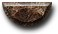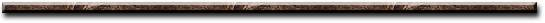News Science Technology Contact Index
 Accelerometer Acceleration is the amount of force needed to move a unit of mass. . What is an accelerometer? An accelerometer is an electromechanical device that measures acceleration forces. It senses how much a mass presses on something when a force acts on it. These forces may be static, like the constant force of gravity pulling at your feet, or they could be dynamic - caused by moving or vibrating. Types of accelerometers There are many different types of accelerometers. The mechanical ones are a bit like scaled-down versions of passengers sitting in cars shifting back and forth as forces act on them. In piezoresistive accelerometers, the mass is attached to a potentiometer (variable resistor), a bit like a volume control, which turns an electric current up or down according to the size of the force acting on it Capacitive accelerometers measure a force in a similar way. When a moving mass alters the distance between two metal plates, measuring the change in their capacitance gives a measurement of the force that's acting. In piezoelectric accelerometers, piezoelectric crystals such as quartz do the clever work. You have a crystal attached to a mass, so when the accelerometer moves, the mass squeezes the crystal and generates a tiny electric voltage. In Hall-effect accelerometers, a mass is unattached and free to move, like a ball in a cup, when the accelerometer moves force is measured by sensing tiny changes in a magnetic field. Semiconductor accelerometers have an electrical terminal with enough mass to move up and down very slightly when you move or tilt the accelerometer - a bit like a bell ringer. The electrical terminal sits between two electrodes separated by an air gap. The air gap between the two electrodes work together as a capacitors. Moving the electrical terminal alters the distances between the electrodes, measuring the changes in their capacitance gives a measurement of the force that's acting on it. Accelerometer Output Analog vs digital - First and foremost, you must choose between an accelerometer with analog outputs or digital outputs. This will be determined by the hardware interfacing with the accelerometer. Analog style accelerometers output a continuous voltage that is proportional to acceleration. E.g. 2.5V for 0g, 2.6V for 0.5g, 2.7V for 1g. Digital accelerometers usually use pulse width Pulse Width Modulation (PWM) for their output. This means there will be a square wave of a certain frequency, and the amount of time the voltage is high will be proportional to the amount of acceleration. Accelerometer Parameters Sensitivity - The more sensitivity the better. This means that for a given change in acceleration, there will be a larger change in signal. Since larger signal changes are easier to measure, you will get more accurate readings. Bandwidth - This means the amount of times per second you can take a reliable acceleration reading. For slow moving tilt sensing applications, a bandwidth of 50Hz will probably suffice. If you intend to do vibration measurement, or control a fast moving machine, you will want a bandwidth of several hundred Hz. Impedance/buffering issues - This is by far the single most common source of problems in projects involving analog accelerometers. Data sheets specify that for A-D conversion to work properly, the connected device must have an output impedance under 10kO. Unfortunately, analog accelerometers have an output impedance of 32kO. The solution to this is to use a low input offset rail to rail op amp as a buffer to lower the output impedance. Accelerometer Applications By measuring the amount of static acceleration due to gravity, you can find out the angle the device is tilted at with respect to the earth. By sensing the amount of dynamic acceleration, you can analyze the way the device is moving. This is useful in cell phone, drone and robotic applications. *** WARNING ACCELEROMETERS CAN BE HACKED *** Resonant sound waves can induce fake motion into capacitive accelerometers. This make them vulnerable to hacking. This poses a serious security threat because most devices inherently trust all the data coming from accelerometers and don't feature protection systems to detect spoofed signals coming from local sensors. The principle behind how the attack works is simple. Accelerometers have a sensing mass that sits on a series of springs. By sending finely-tuned sound waves to the accelerometer, the sound waves induce controlled movement into sensing mass. Sound waves used in these attacks could be embedded in songs played over a user's speakers. No high-end speakers are needed, and regular \$5 speakers could be used to replay the malicious sound waves.[News] [Science] [Technology] [Contact] [Index]
 Contact email: qooljaq@qooljaq.com